## Example Questions

### Example Question #1 : How To Find An Angle In A Hexagon

Quantitative Comparison

Quantity A: The degree measure of any angle in an equilateral triangle

Quantity B: The degree measure of any angle in a regular hexagon

Quantity A is greater.

The two quantities are equal.

The relationship cannot be determined from the information given.

Quantity B is greater.

Quantity B is greater.

Explanation:

We know the three angles in a triangle add up to 180 degrees, and all three angles are 60 degrees in an equilateral triangle.

A hexagon has six sides, and we can use the formula degrees = (# of sides – 2) * 180. Then degrees = (6 – 2) * 180 = 720 degrees. Each angle is 720/6 = 120 degrees.

Quantity B is greater.

### Example Question #2 : How To Find An Angle In A Hexagon

Quantity A: Double the measure of a single interior angle of an equilateral triangle.

Quantity B: The measure of a single interior angle of a hexagon.

The quantities are equal.

Quantity A is bigger.

The relationship cannot be determined with the information given.

Quantity B is bigger.

The quantities are equal.

Explanation:

Begin with Quantity A. We know the measure of one angle in an equilateral triangle is 60. Therefore, double the angle is 120 degrees.

For the hexagon, use the formula for the sum of the interior angles: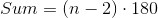where n= number of sides in a regular polygon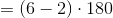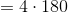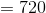If the sum of the interior angles of a regular hexagon is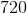degrees, then one angle is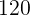degrees.

The two quantities are equal.

Tired of practice problems?

Try live online GRE prep today.